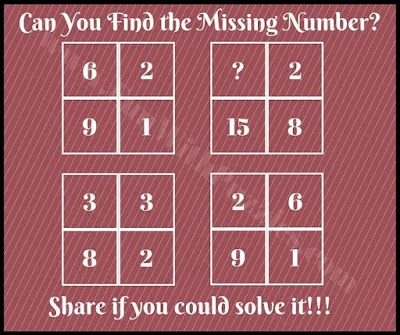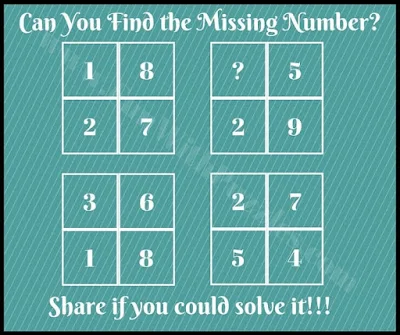Here are some Maths Brain Twister Questions. In the Math Brain Teasers put some numbers together which relate to each other with some mathematical operations. Sometimes it becomes very confusing to find this relationship and then solve it for the missing number. Let us see if you can solve such puzzles quickly.

## How to Solve Easy Maths Brain Teasers Questions

In these puzzles, four squares are given with each square having four numbers. Numbers given in these squares relate to each other with some mathematical formulas. Your Math challenge is to find these Maths formulas which relate to these numbers and then solve them for the missing number.

Answers to these Math Brain Twister Questions are given at the end. Do write your answers in the comments section of this post with your reasoning to solve these brain teasers.1. Can you solve this easy Maths Brain Teaser?2. Can you solve this fun Maths Brain Teaser?4. Can you find the Missing Number in 3rd Square?5. Can you find the Missing Number in the 2nd Square?

Check out similar Puzzles and Brain Teasers as mentioned below to twist your brain

## List of Puzzles, Riddles, and Brain Teasers

1. Matchstick Math Brain Teasers for Teens with Answers: If you love to solve Math Puzzles then you will definitely fall in love with these Matchstick Math Brain Teasers.

2. Mind-Blowing Math Puzzle Questions: These are very tough Maths Puzzles to Blow your mind.

3. Interesting Quick Riddles with Answers: These are very interesting quick Riddles that you can ask your friends.

4. Learn to solve "Spot the Difference Picture Puzzles" quickly: After solving all these Math Brain Teasers and Riddles, now it is time to learn to solve "Spot the Difference Picture Puzzles" very quickly.

Answers to these Math Brain Twister Questions are given below. Try to find solutions to all these brain teasers yourself without looking at these answers. Do use these answers to check your solution.

## Answers to Easy Maths Brain Teasers Questions

A1. 5

A2. 4

A3. 6

A4. 5

A5. 2

Unknown said...

3

Unknown said...

2

Anonymous said...